## How to get the current time in millisecond using JavaScript?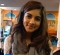Published on 16-Feb-2018 08:03:27
To get the current time in a millisecond, use the date getMilliseconds() method. JavaScript date getMilliseconds() method returns the milliseconds in the specified date according to local time. The value returned by getMilliseconds() is a number between 0 and 999.Example You can try to run the following code to get the ... Read More

## How to convert a JavaScript date object to a string?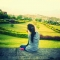Published on 16-Feb-2018 08:00:38
To convert a JavaScript date object to a string, use the toString() method. This method returns a string representing the specified Date object.ExampleYou can try to run the following code to convert a date object to a string −<html>    <head>       <title>JavaScript toString() Method</title>    </head>   ... Read More

## How to get the value of a number rounded to the nearest integer in JavaScript?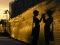Published on 16-Feb-2018 07:56:48
Use the Math.round() method to get the value of a number rounded to the nearest integer.ExampleYou can try to run the following code to return the value of a number rounded to the nearest integer in JavaScript −<html>    <head>       <title>JavaScript Math round() Method</title>    </head>   ... Read More

## How to get the exponent power of a number in JavaScript?Published on 16-Feb-2018 07:58:25
To get the exponent power of a number, use the Math.pow (base, exponent ) method. This method returns the base to the exponent power, that is, base exponent. The following are the parameters used in Math.pow() method −base − The base number. exponents − The exponent to which to raise base.ExampleYou can try to run ... Read More

## How to calculate the area and perimeter of a circle in JavaScript?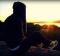Published on 16-Feb-2018 07:50:50
To calculate the area and perimeter of a circle, you can try to run the following code −Example<html>    <head>       <title>JavaScript Example</title>    </head>    <body>       <script>          function Calculate(r) {             this.r = r;   ... Read More

## How to create RegExp object in JavaScript?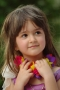Published on 16-Feb-2018 07:50:40
A regular expression is an object that describes a pattern of characters. The JavaScript RegExp class represents regular expressions, and both String and RegExp define methods that use regular expressions to perform powerful pattern-matching and search-and-replace functions on the text.A regular expression could be defined with the RegExp () constructor, ... Read More

## What are regular expressions in JavaScript?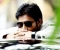Published on 16-Feb-2018 07:44:55
A regular expression is an object that describes a pattern of characters. The JavaScript RegExp class represents regular expressions, and both String and RegExp define methods that use regular expressions to perform powerful pattern-matching and search-and-replace functions on the text.A regular expression could be defined with the RegExp () constructor, as ... Read More

## How to convert a negative number to a positive one in JavaScript?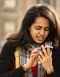Published on 16-Feb-2018 07:43:59
To convert a negative number to a positive one in JavaScript, use the abs() method in JavaScript. The method returns the absolute value of a number.ExampleYou can try to run the following code to convert a negative number to positive −<html>    <head>       <title>JavaScript Math abs() Method</title> ... Read More

## What are C++ Character Constants?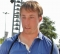Published on 16-Feb-2018 07:32:13
Character constants are one or more members of the “source character set, ” the character set in which a program is written, surrounded by single quotation marks ('). They are used to represent characters in the “execution character set, ” the character set on the machine where the program executes. ... Read More

## How to do basic Maths with JavaScript Operators?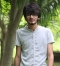Published on 16-Feb-2018 07:34:48
To do basic Maths such as +, -, * in JavaScript, use the Operators. JavaScript includes operators for basic mathematics such as Addition, Subtraction, Multiplication, etc.ExampleYou can try to run the following code to learn how to work with basic Maths i.e. Mathematical Operators in JavaScript −<html>    <body>   ... Read More